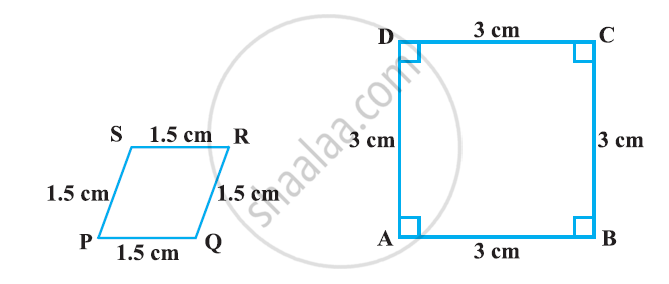# State Whether the Following Quadrilaterals Are Similar Or Not - Mathematics

State whether the following quadrilaterals are similar or not:#### Solution

Quadrilateral PQRS and ABCD are not similar as their corresponding sides are proportional, i.e. 1:2, but their corresponding angles are not equal.

Concept: Similar Figures
Is there an error in this question or solution?
Chapter 6: Triangles - Exercise 6.1 [Page 122]

#### APPEARS IN

NCERT Class 10 Maths
Chapter 6 Triangles
Exercise 6.1 | Q 3 | Page 122

Share